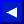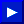In this example you used N to remind yourself that this variable stands for "NAME". The dollar sign (\$) is used to tell the computer that you're using a string variable. It is important to differentiate between the two types of variables:

1. NUMERIC
2. STRING

You probably remember from the earlier sections that numeric variables are used to store number values such as 1, 100, 4000, etc. A numeric variable can be a single letter (A), any two letters (AB), a letter and a number (AI), or two letters and a number (AB1). You can save memory space by using shorter variables. Another helpful hint is to use letters and numbers for different categories in the same program (AI, A2, A3). Also, if you want whole numbers for an answer instead of numbers with decimal points, all you have to do is put a percent sign (%) at the end of your variable name (AB%, AI%, etc.) Now let's look at a few examples that use different types of variables and expressions with the INPUT statement.

```   10 PRINT"ENTER A NUMBER": INPUT A
20 PRINT A

10 PRINT"ENTER A WORD": INPUT A\$
20 PRINT A\$

10 PRINT"ENTER A NUMBER": INPUT A
20 PRINT A "TIMES 5 EQUALS" A*5
```

 NOTE: Example 3 shows that MESSAGES or PROMPTS are inside the quotation marks (" ") while the variables are outside. Notice, too, that in line 20 the variable A was printed first, then the message "TIMES 5 EQUALS", and then the calculation, multiply variable A by 5 (A*5).

Calculations are important in most programs. You have a choice of using "actual numbers" or variables when doing calculations, but if you're working with numbers supplied by a user you must use numeric variables. Begin by asking the user to type in two numbers like this:

```   10 PRINT"TYPE 2 NUMBERS": INPUT A: INPUT B
```This page has been created by Sami Rautiainen. Read the small print. Last updated November 14, 1998.